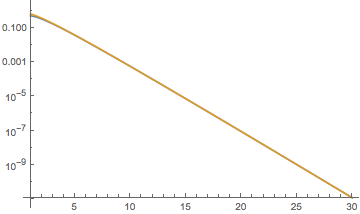# Asymptotic behavior of integral with gamma functions

Consider the following function defined for complex numbers $z\in\mathbb{C}$ with $\Re(z)\geq \frac{1}{2}$:

$$F(z)=\frac{1}{5^{\Re(z)}}\int_0^\infty \left| \frac{\Gamma(z+ix)\Gamma(z-ix)}{\Gamma(z)^2} \cdot \exp\left( \frac{\pi}{2} x\right)\right| dx.$$

I am wondering about the behavior of $F(z)$ for $\vert z \vert \rightarrow \infty$.

• How is $z$ tending to infinity? $\Re(z) \to \infty$? Or $|z| \to \infty$? Or is it tending to infinity in some sector of the complex plane? – user78249 Apr 15 '17 at 15:50
• z is tending to infinity in the domain of definition. That is $\vert z \vert\rightarrow \infty$ with $\Re(z)\geq 1/2$ – Sammyy Delbrin Apr 15 '17 at 16:19

If I just insert the large-$z$ asymptotics of $\Gamma(z)\rightarrow \sqrt{2 \pi } e^{-z} z^{z-\frac{1}{2}}$, and take $z>1/2$ real for simplicity, I find $$5^z\,F(z)\rightarrow \int_0^\infty \left(1+x^2/z^2\right)^{z-\frac{1}{2}} e^{\pi x/2-2 x \arctan \left(x/z\right)}\,dx$$ $$\qquad = z\int_0^\infty(1+x^2)^{-1/2}\exp[z u(x)]\,dx$$ $$\text{with}\;\;u(x)=\pi x/2-2x\arctan x+\ln(1+x^2).$$ This is readily evaluated in the large-$z$ limit by steepest descent. Since $u'(x)=\frac{1}{2}(\pi-4\arctan x)$, the maximum of $u(x)$ is at $x=1$, so we expand $$u(x)=\ln 2-\tfrac{1}{2}(1-x)^2+\cdots,$$ and carry out the Gaussian integral, to arrive at $$F(z)\rightarrow (2/5)^{z}\sqrt{\pi z}.$$ Here is a numerical test, blue the exact $F(z)$ and gold the asymptote:the agreement is very precise (the two curves are pretty much indistinguishable).

The steepest descent for complex $z$ can be carried out in the same way, but is a more tedious calculation.

The Mathematica 11.1.0.0 command

Series[Abs[(E^((\[Pi] x)/2) Gamma[-I x + z] Gamma[I x + z])/
Gamma[z]^2], {x, Infinity, 3}, Assumptions -> x > 0]


outputs $$\frac{\exp \left(\Re\left(-\pi x+(\log (2 \pi )+2 z \log (x)-\log (x))+\frac{2 z^3-3 z^2+z}{6 x^2}+O\left(\left(\frac{1}{x}\right)^3\right)\right)+\frac{\pi x}{2}\right)}{\left| \Gamma (z)\right| ^2} .$$

Hope this would be useful.

Addition. The asymptotics of the integrand as $z \to \infty$ can be found as follows.

Series[Abs[(E^((\[Pi] x)/2) Gamma[-I x + z]*Gamma[I x + z])/
Gamma[z]^2], {z, ComplexInfinity, 2}, Assumptions -> x > 0]


Because I have a problem with inserting its output in $\LaTeX$ form, the one may be submitted through Dropbox on demand. The output is neither simple nor nice.

Table[Log[1/5^Re[z]* NIntegrate[Abs[(E^((\[Pi] x)/2)* Gamma[-I x + z]*Gamma[I x + z])/
Gamma[z]^2], {x, 0, Infinity}]], {z, 100, 400, 100}]


performs a warning and $\{-88.7554,-180.037,-271.463,-362.949\}$ and

Table[Log[1/5^Re[z]* NIntegrate[Abs[(E^((\[Pi] x)/2)* Gamma[-I x + z]*Gamma[I x + z])/
Gamma[z]^2], {x, 0, Infinity}]], {z, 20 + 100*I, 20 + 400*I, 100*I}


produces a warning and $\{89.3217,232.976,382.167,533.642\}$.

Addition 3. The plot produced by

Plot3D[Log[(1/5^Re[z] /. z -> u +I*v)*NIntegrate[ Abs[(E^((Pi*x)/2)*
Gamma[-I *x + z]*Gamma[I* x + z])/Gamma[z]^2] /. z -> u + I*v, {x, 0, Infinity},
Method -> "GlobalAdaptive", AccuracyGoal -> 4, WorkingPrecision -> 15]], {u, -50, 50},
{v, -50, 50},AxesLabel -> Automatic]suggests the dependence of the integral under consideration on $u=\Re z$ mostly.

Too long for a comment:

By using the approach of @Carlo Beenakker I was able to get an asymptotic formula for $z=\frac{1}{2} + z_{0} e^{i \phi}$ with $z_{0}\rightarrow\infty$ and $-\frac{\pi}{2}<\phi<\frac{\pi}{2}$. Here it is: $$F(z) \sim \left(\frac{2}{5}\right)^{\Re (z)} \sqrt{\pi z_0 \cos \phi} \exp\left\{z_0 \left[\phi \ \sin{\phi}+\cos{\phi} \ln{(\cos{\phi})}\right]\right\}.$$ Near the limits of the validity interval for $\phi$ the approximation breaks down. For $\phi = \pm\frac{\pi}{2}$ and $z_0 \rightarrow \infty$ I found $$F(\frac{1}{2} \pm i z_{0})\sim \frac{2}{\pi\sqrt{5}}\left(2\ e^{z_{0}\frac{\pi}{2}}-1\right).$$

Edit: However, in the last formula a factor is missing. But for the asymptotic case $z=\frac{1}{2} + i z_{0}$ with $z_{0}\rightarrow \infty$ it is anyway easier to exploit the identity $$\left|\Gamma\left(\frac{1}{2} + i y\right)\right|^2 = \frac{\pi}{\cosh (\pi y)}.$$ After inserting in the definition of $F(z)$ and some variable transformations on gets $$\sqrt{\frac{2}{5}}\frac{\beta+\beta^{-1}}{2^{3/2}\pi}\int_{1}^{\infty} dz \ z^{-1/4} \left(z^2 +(\beta+\beta^{-1})z+1\right)^{-1/2}$$ with the abbreviation $\beta = e^{\pi z_{0}}$. Mathematica can solve this integral in terms of Gaussian Hypergeometric functions and an Appell function, $F_{1}$ (see for example here). This can be expanded for large $z_{0}$ $$F\left(\frac{1}{2}+ i z_{0}\right) = \sqrt{\frac{2}{5}} \frac{\Gamma\left(\frac{1}{4}\right)^2}{(2 \pi)^{3/2}} e^{\frac{\pi}{2}z_{0}}+O(e^{-\frac{\pi}{2}z_{0}})$$